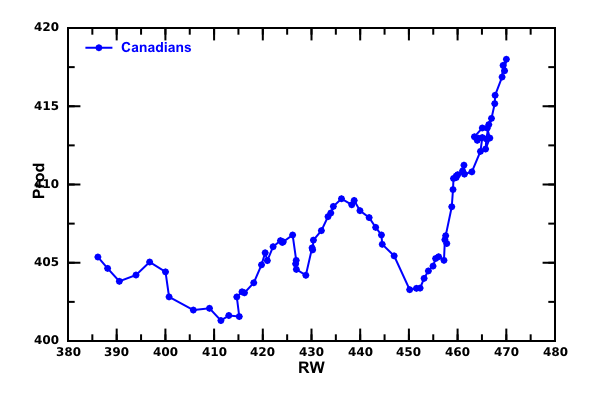# DMelt:Finance/Time Series

Limitted access. First login to DataMelt if you are a full DataMelt member. Then login to HandWiki as a user.

# Time series

DataMelt can read data (time series) in variety of formats, such as ASCII, Gauss and Matlab. One can read and write data in Microsoft Excel 97 formats (the extension "xls"). Data can be modified, showed as tables, plotted. Also, a statistical analysis can be performed. One can also save such data into ASCII, Gauss, Matlab,

The calculations with time series are based on the classHStatData. Let us read data written in one of the popular formats, such as ASCII, Gauss, Matlab, Excel.

from jhpro.tseries import *
js=HStatData("Data","http://datamelt.org/examples/data/jhpro/tseries/asciitest1.dat")
js.toTable()

You will see a table with time series populated with the data. One can save this table back using "saveData" method:

from jhpro.tseries import *
js=HStatData("Data","http://datamelt.org/examples/data/jhpro/tseries/asciitest1.dat")
js.saveData("data.dat","txt")

We have specified "txt" (ASCII) format.One can also save data in Gauss, Matlab and Excel by specifying the appropriate string. ReadHStatData class description.

For example, try the following methods:

js.saveData("aaa.mat","matlab")
js.saveData("aaa.xls","Excel")
js.saveData("aaa.gauss","GaussDat")

You can also convertHStatData tojhplot.PND and use the methods of PND. Finally, you can serialize HStatData into a compressed file, or XML using the Serialized class (see man:io:input_output).

You can read a number of example files into the time-series class. DMelt supports many file formats. Examples of supported files are located in the financial file format directory.

# Time series data formats

Data for time series are represented using the JMulti convention.

In case of ASCII data file, the file will look as:

/∗seasonally adjusted, West Germany: fixed investment, disposable income, consumption expenditures ∗/
183 451 412
174 462 422
...
...

The file should contain the data of each variable in a column, while missing values may be coded with NaN. The comment is optional.

There is another form of JMulTi which allows for easy data recognition without further user interaction. The following is an example of a ".dat" file with an optional description:

/∗seasonally adjusted, West Germany: fixed investment, disposable income, consumption expenditures ∗/
3 1960.1 4
invest income cons
180 451 415
179 465 421
...
...

where the first number defines the number of variables. The second number is the start date, and the last number the periodicity of the data set. The start date must be a valid date for the given periodicity. For example, 1960.1 stands for the first quarter of 1960 because 4 defines quarterly data. Yearly data has periodicity 1. The periodicity can be chosen to be any positive integer. It should be noticed that, for monthly data, January is coded with 1960.01, whereas 1960.1 or 1960.10 stands for October 1960.

# Plotting time series

Plotting of time series is not too difficult, assuming that you know how to work with jHPlot (or any other) canvases. You can convert 2 columns of data into the P1D class and use it to draw X vs Y:

Here is a result of the output of the above code which reads time series:Often, you would like to replace the labels of the X-axis so they will show the actual time. This trick was discussed in man:visual:plot_styles. Below you can find an example which makes the actual replacement:

# Descriptive statistics

One can do a full-scale analysis of time series using many powerful methods described below. Here is a 6-line Python macro which extracts one column from a data series and performs a detailed statistical analysis:

The output of this short script is given below. As you can see, it prints mean,. RMS, variance, Standard deviation, min and max values, Skewness, kurtosis and high order moments:

Size: 88
Sum: 79317.60942234722
SumOfSquares: 7.490318884164342E7
Min: 0.0
Max: 961.765709811429
Mean: 901.3364707084911
RMS: 922.5901584523979
Variance: 39210.743676671525
Standard deviation: 198.0170287542754
Standard error: 21.10868619051051
Geometric mean: 0.0
Product: 0.0
Harmonic mean: 0.0
Sum of inversions: Infinity
Skew: -4.275748299773066
Kurtosis: 16.51526905197178
Sum of powers(3): 7.074101996131584E10
Sum of powers(4): 6.681632756449685E13
Sum of powers(5): 6.3115221764760856E16
Sum of powers(6): 5.962464385763685E19
Moment(0,0): 1.0
Moment(1,0): 901.3364707084911
Moment(2,0): 851172.6004732207
Moment(3,0): 8.038752268331345E8
Moment(4,0): 7.592764495965552E11
Moment(5,0): 7.172184291450098E14
Moment(6,0): 6.7755277110950963E17
Moment(0,mean()): 1.0
Moment(1,mean()): -5.6843418860808015E-14
Moment(2,mean()): 38765.16704398216
Moment(3,mean()): -3.3198598540862594E7
Moment(4,mean()): 3.0004383082685673E10
Moment(5,mean()): -2.704012982455758E13
Moment(6,mean()): 2.4372448867975816E16
25%, 50%, 75% Quantiles: 933.6877992686658, 945.85509828759, 949.9494485185958
quantileInverse(median): 0.5056818181818115

# Time series analysis

Time series can be analyzed in many different approaches by extraction columns and rows of the data. In particular, you can construct autocorrelation and cross-correlation vectors. and plot them. One can also perform Gaussian filtering and detect peaks using a peak finder algorithm.

# Time series transformations

Time series can be transformed using an analytic functions. Essentially, you can construct a function of any complexity using functions using the same syntax as for 1D functionsjhplot.F1D. Find below a stript which transforms the first column of the time series container using the function $1+\sqrt(x)$

# Histograms

To show an column as a histogram is a convenient way to sudy the properties of time series. Below we show how to convert a column tojhplot.H1D histogram and show it on the canvas:

The output of this code is shown below.